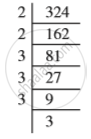# Square Roots - Finding Square Root Through Prime Factorisation

#### description

• Prime factorisation of a Number, Prime factorisation of its Square

#### notes

Consider the prime factorisation of the following numbers and their squares.

 Prime factorisation of a Number Prime factorisation of its square 6 = 2 × 3 36 = 2 × 2 × 3 × 3 8 = 2 × 2 × 2 64 = 2 × 2 × 2 × 2 × 2 × 2 12 = 2 × 2 × 3 144 = 2 × 2 × 2 × 2 × 3 ×3 15 = 3 × 5 225 = 3 × 3 × 5 × 5

You will find that each prime factor in the prime factorisation of the square of a number, occurs twice the number of times it occurs in the prime factorisation of the number itself.
Let us use this to find the square root of a given square number, say 324.
We know that the prime factorisation of 324 is
324 = 2 × 2 × 3 × 3 × 3 × 3By pairing the prime factors, we get
324 =2 × 2 × 3 × 3 × 3 × 3
= 2^2 × 3^2 × 3^2 = (2 × 3 × 3)^2
So,sqrt 324 =2 × 3 × 3 = 18

If you would like to contribute notes or other learning material, please submit them using the button below.